Equable Triangle

EQUABLE TRIANGLE
and related topics

Balmoral Software

Solutions: 5

### Problem Definition

This paper discusses cases where the area A of a Heronian triangle (HT) is a rational factor t times its perimeter P:
 A = tP, 1 ≤ t ≤ 3, 
so that t = 1 corresponds to the equable case. If t > 1, it is assumed to be of the form t = h/(h - 2) for some integer h ≥ 3, and will be used to determine equable triangular prisms. In a later section, other results are presented for cases where the perimeter of an HT exceeds its area (t < 1).

As was proven over a century ago, exactly five HTs are equable [A]:

SidesPerimeter = Area
(10,8,6) *24
(13,12,5) *30
(17,10,9)36
(20,15,7)42
(29,25,6)60

*: Right triangle

Following is a proof of the results for the general relation  that includes the five equable triangles as a particular case.

Let the integer sides of an HT be a, b, c, and denote by s its semiperimeterBy the Triangle Inequality,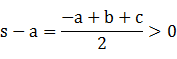and analogously, s - b > 0 and s - c > 0. Using Heron's area formula,
 A2 = s(s - a)(s - b)(s - c) 

16A2 = P(P - 2a)(P - 2b)(P - 2c)

If the perimeter P = 2s is odd, then s is of the form k + 1/2 for some positive integer k, and so is each of the four factors in A2, whence the product is of the form m + n/16 for positive integers m and n, 1 < n < 16. Therefore, the area and the sides cannot all be integers if P is odd. Henceforth, we will assume that the perimeter is even so that the area is an integer. It follows that the semiperimeter s is also an integer.

Without loss of generality, we can assume that the integer sides of an HT are ranked alphabetically from longest to shortest:

 1 ≤ c ≤ b ≤ a < b + c 

### Change of Variables

To simplify the algebra, we introduce new integers x, y, and z:
 x = s - a  y = s - b  z = s - c 
These integers are all strictly positive by the previous results. Summing both sides of the equations above, we have
 x + y + z = 3s - (a + b + c) = 3s - 2s = s 
The square of the HT area can now be written more simply as
 A2 = sxyz 
The inverse relationships between x, y, z and the sides of the triangle are:
 a = s - x = y + z b = s - y = x + z c = s - z = x + y 
Substituting variables in , we have
 1 ≤ x ≤ y ≤ z 
The variables in the preceding inequality are unbounded, so to find all HTs satisfying , we will determine limits for x, y and z. All solutions can then be found by a computer-aided search over a finite and manageable set of possibilities.

### Limits of Variables

By ,  and , we have
 xyz = 4t2(x + y + z)  (xy - 4t2)z - 4t2(x + y) = 0 
First, assume x ≥ 36. Then by , we have
yz ≥ x2 ≥ 362 > 36 ≥ 4t2

yz - 4t2 > 0

Since x ≥ 4t2, we can write  as
4t2(y + z) = x(yz - 4t2) ≥ 4t2(yz - 4t2)
It follows that
 (y - 1)(z - 1) ≤ 4t2 + 1 ≤ 37 
But
(y - 1)(z - 1) ≥ (x - 1)2 ≥ 352 = 1225,
which contradicts . Therefore, x ≤ 35:
xyxyzSolutions to A = tP
x ≥ 36anyanyanynone
1 ≤ x ≤ 35anyanyanyTBD
Next, assume x ≤ 35 and y ≥ 87. Then
(y - 36)2 > 2556
Since z ≥ y, we have
(y - 36)(z - 36) > 2556

yz > 36(y + z + 35)

Since 1 ≤ x ≤ 35 and 4t2 ≤ 36, it follows that
xyz > 4t2(x + y + z),
so there are no solutions to  when x ≤ 35 and y ≥ 87:
xyxyzSolutions to A = tP
x ≥ 36anyanyanynone
1 ≤ x ≤ 35y ≥ 87anyanynone
1 ≤ x ≤ 35x ≤ y ≤ 86anyanyTBD
Note that if xy - 4t2 ≤ 0, then
(xy - 4t2)z - 4t2(x + y)
is negative, and there is no solution to :
xyxyzSolutions to A = tP
x ≥ 36anyanyanynone
1 ≤ x ≤ 35y ≥ 87anyanynone
1 ≤ x ≤ 35x ≤ y ≤ 86xy ≤ 4t2anynone
1 ≤ x ≤ 35x ≤ y ≤ 86xy > 4t2anyTBD
Henceforth, assume x ≤ 35, x ≤ y ≤ 86 and xy > 4t2. If 4t2 ∈ ℤ, then xy ≥ 4t2 + 1, but if 4t2 ∉ ℤ, then
xy ≥ ⌈4t2⌉ = ⌊4t2⌋ + 1,
where ⌈⌉ and ⌊⌋ are the ceiling and floor functions, respectively. Thus, in all cases,
 xy ≥ ⌊4t2⌋ + 1 
From the prism convention in the Introduction, we have
1/289 ≤ ⌊4t2⌋ - 4t2 + 1 ≤ 1
or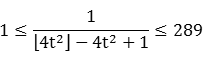Let z > 1,258,884. Then(⌊4t2⌋ - 4t2 + 1)z > 4t2(x + y)

(xy)z ≥ (⌊4t2⌋ + 1)z > 4t2(x + y + z)

by , so there is no solution to :
xyxyzSolutions to A = tP
x ≥ 36anyanyanynone
1 ≤ x ≤ 35y ≥ 87anyanynone
1 ≤ x ≤ 35x ≤ y ≤ 86xy ≤ 4t2anynone
1 ≤ x ≤ 35x ≤ y ≤ 86xy > 4t2z > 1,258,884none
1 ≤ x ≤ 35x ≤ y ≤ 86xy > 4t2y ≤ z ≤ 1,258,884TBD

### Enumeration of Solutions

Now that we have found finite bounds for all variables x, y and z, searching for HTs for which  is satisfied is a manageable problem that can be solved on a computer in a few minutes. At the expense of finding and discarding a relatively few extraneous results when xy ≤ 4t2, we can ignore the restriction on xy and focus solely on the finite bounds for x, y and z. The number of outcomes to be considered then is: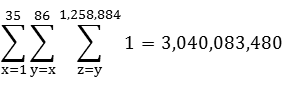For each of these possibilities, the following steps will be completed:
Compute s = x + y + z and P = 2s
Verify that A2 = sxyz is a square
If A = P, output results for equable triangles
If A > P and 2A/(A - P) is an integer, output results for equable triangular prisms
The five equable HTs (t = 1) are listed below, in order of increasing area:
 a b c A=P x y z Triangle type 10 8 6 24 2 4 6 Right 13 12 5 30 2 3 10 Right 17 10 9 36 1 8 9 Obtuse 20 15 7 42 1 6 14 Obtuse 29 25 6 60 1 5 24 Obtuse

### Plots

For display purposes, we will follow the convention of orienting a triangle with its longest side a along the horizontal x-axis with the origin at the left endpoint, and the other sides b and c arranged clockwise in decreasing order. The general form of the triangle then isBy the distance formula,
 u2 + v2 = b2  (u - a)2 + v2 = c2
Subtracting both sides,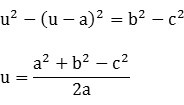From  and A = P, we have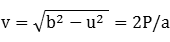The inradius of the triangle is
r = A/s = P/s = 2
Since the base of the triangle lies on the x-axis and the incircle is tangent to it, the y-coordinate of the incenter is also 2. Therefore, the incenter is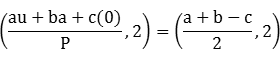The circumcircle of this triangle passes through the non-collinear vertices (0,0), (a,0) and (u,v). Its circumcenter can be found by the method of determinants. LetThen the circumcenter is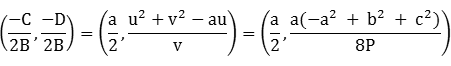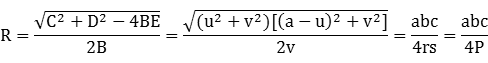Evaluating the formulas for the vertex coordinates and the inscribed and circumscribed circles of each equable HT, we have
108624(10,0)(32/5,24/5)2(6,2)5(5,0)
1312530(13,0)(144/13,60/13)2(10,2)13/2(13/2,0)
1710936(17,0)(154/17,72/17)2(9,2)85/8(17/2,-51/8)
2015742(20,0)(72/5,21/5)2(14,2)25/2(10,-15/2)
2925660(29,0)(715/29,120/29)2(24,2)145/8(29/2,-87/8)
The results above can be used to create scale drawings of the five equable HTs and their corresponding incircles and circumcircles:### Small Heronian Triangles

It is well known that no HT can have a side of length 1 or 2. To see this, let (a,b,1) be the integer sides of an HT. By the triangle inequalities,
a < b + 1 and b < a + 1
or
b - 1 < a < b + 1
This is satisfied only when a = b, in which case the perimeter 2a + 1 is odd, and therefore the triangle cannot be Heronian.

Next, let (a,b,2) be the integer sides of an HT. Again applying the triangle inequalities,

b - 2 < a < b + 2
or
b - 1 ≤ a ≤ b + 1
If a is b ± 1, then the perimeter (b ± 1) + b + 2 is again odd.

The remaining possibility is the isosceles triangle (b,b,2), in which case the semiperimeter s is b + 1 and by , the square of the area is

A2 = (b + 1)(1)(1)(b - 1)

b2 - A2 = 1,

which has no solution in integers since the difference of two squares cannot be 1.

### Perimeter-dominant Triangles

Consider integer-sided (not necessarily Heronian) triangles with perimeter greater than area. The following results will be established for these perimeter-dominant triangles, based on the shortest triangle side:
CaseShortest
side c
xyNumber of perimeter-dominant
integer-sided triangles
1c ≥ 17anyNone
21 ≤ c ≤ 16xy > 4317
31 ≤ c ≤ 16xy ≤ 4Infinitely many
Case 1

In , apply a lower bound to the integer triangle sides:

 17 ≤ c ≤ b ≤ a < b + c, 
We'll use the same variables s, x, y and z defined in , ,  and , but won't restrict the analysis to triangles with even perimeters, so s may be an integer or an odd integer multiple of 1/2. The sum of any two of {x,y,z} must be an integer since it is equal to one of the triangle sides, so {x,y,z} are either all integers or all odd multiples of 1/2. Equivalently, the doubles {2x,2y,2z} all have the same parity. The constraints in  may be written
1/2 ≤ x ≤ y ≤ z and x + y ≥ 17
We can write
 17 - y ≤ x ≤ y z ≥ y ≥ 17/2 
Perimeter dominance requires that
 P2 = (2s)2 > s(s - a)(s - b)(s - c) = A2 4(x + y + z) > (s - a)(s - b)(s - c) = xyz  (xy - 4)z < 4(x + y) 
Note that for x < 3, we have
 x 17 - x >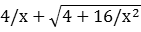0.5 16.5 > 16.246 1 16 > 8.472 1.5 15.5 > 6 2 15 > 4.828 2.5 14.5 > 4.161
so that(y - 4/x)(z - 4/x) ≥ (y - 4/x)2 > 4 + 16/x2

xyz > 4y + 4z + 4x

which contradicts . We henceforth take x ≥ 3, so that xy ≥ 9. Then
(x - 8/17)2 > 1220/289

(x - 8/17)(y - 8/17) > 4 + 64/289

17xy - 8x - 8y > 68

4x + 4y < (17/2)xy - 4(17/2)

Dividing by xy - 4 ≥ (3)(3) - 4 > 0 and using , we have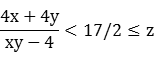(xy - 4)z > 4x + 4y

which contradicts . We conclude that there are no perimeter-dominant integer-sided triangles when c ≥ 17.

Case 2

For 1 ≤ c ≤ 16, first assume xy > 4 so that we can perform division in :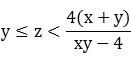which by  reduces to the cubic
 y3 - cy2 + 4y + 4c > 0 
Since 1/2 ≤ x ≤ y = c - x, it follows that
 c/2 ≤ y ≤ c - 1/2 
To determine all the triangle solutions, we simply iterate over integers c such that 1 ≤ c ≤ 16, and half-integers y satisfying . For each of the 136 resulting combinations of c and y, we set x = c - y, determine if xy > 4 and  are satisfied, and if so, apply the limits for z in  such that the double of z has the same parity as the doubles of x and y. There are a total of 317 solutions when 5 ≤ c ≤ 16 and xy > 4, listed here and summarized below:
Shortest
side c
Number of perimeter-dominant
integer-sided triangles (xy > 4)
527
630
710
84
9137
1044
1125
1216
1311
147
154
162
Total317
For example, if (x,y,z) = (0.5,12.5,17.5), the corresponding integer triangle (a,b,c) = (30,18,13) has perimeter 61 that is greater than its area 57.758. There aren't any solutions for 1 ≤ c ≤ 4 since that implies xy ≤ 4.

Case 3

Now assume 1 ≤ c ≤ 16 and xy ≤ 4. We have

1/2 ≤ x ≤ y ≤ 4/x,
so x has only the four values
1/2 ≤ x ≤ 2
In , we have
(xy - 4)z < 4(x + y) = 4c
Since xy ≤ 4 and the right side of the inequality above is strictly positive, the two sides bracket 0 regardless of the value of z, and so there are infinitely-many perimeter-dominant integer triangles. We have the following possibilities for x and y since their doubles must have the same parity:
 x 4/x y ≤ 4/x Number of (x,y)combinations c = x + y 0.5 8 0.5,1.5,...,7.5 8 1,2,3,4,5,6,7,8 1 4 1,2,3,4 4 2,3,4,5 1.5 8/3 1.5,2.5 2 3,4 2 2 2 1 4 Total 15
For example, if x = 1 and y = 3, then c = x + y = 4, xy - 4 = -1 and inequality  is written
(-1)z < 4(4),
which is true for any value of z. Since b = a - (y - x), we have the following equivalent representations:
 c (x,y) Triangle forms (a,b,c) 1 (0.5,0.5) (a,a,1)* 2 (0.5,1.5),(1,1) (a,a-1,2), (a,a,2)* 3 (0.5,2.5),(1,2),(1.5,1.5) (a,a-2,3), (a,a-1,3), (a,a,3)* 4 (0.5,3.5),(1,3),(1.5,2.5),(2,2) (a,a-3,4), (a,a-2,4), (a,a-1,4), (a,a,4)* 5 (0.5,4.5),(1,4) (a,a-4,5), (a,a-3,5) 6 (0.5,5.5) (a,a-5,6) 7 (0.5,6.5) (a,a-6,7) 8 (0.5,7.5) (a,a-7,8)
*: Isosceles
One conclusion that can be drawn from these results is that any isosceles triangle with a base of 4 or less has a perimeter greater than its area (this is true whether the triangle sides are integers or not).

### Heronian Perimeter-dominant Triangles

Now consider the subset of perimeter-dominant integer triangles that have integer area (and therefore even perimeters); these perimeter-dominant HTs will be of interest in the analysis of certain pentagons. The following results show that there are infinitely-many perimeter-dominant HTs. Exactly one is Pythagorean, and exactly two are isosceles, each consisting of two back-to-back Pythagorean triangles. The sides of all other perimeter-dominant HTs are of the nearly-isosceles forms (a,a-1,3) or (a,a-2,4).

First, we can scan the results from Case 2 above to find that there is only one perimeter-dominant HT for which xy > 4: the (6,5,5) isosceles triangle with perimeter 16 and area 12. This triangle consists of two back-to-back copies of the smallest primitive Pythagorean triangle (3,4,5).

For Case 3, we remove the odd-perimeter cases from the preceding table to find the following possibilities for a perimeter-dominant HT:

 Triangle forms (a,b,c) (a,a,2) (a,a-1,3) (a,a-2,4) (a,a,4) (a,a-3,5)
(a,a,2) triangles

We have from ,

A2 = (a + 1)(1)2(a - 1)

a2 - A2 = 1,

which has no solution since the difference of two squares cannot be 1.

(a,a,4) triangles

The area formula is

A2 = (a + 2)(2)2(a - 2)

(2a + A)(2a - A) = 16,

The possible factors are
 2a + A 2a - A sum = 4a > 16 > 1 17 8 > 2 10
Since neither of these results in an integer value for a, there is no solution.

(a,a-3,5) triangles

We have

A2 = (a + 1)(1)(4)(a - 4)

(2a - 3 + A)(2a - 3 - A) = 25,

The possible factors are
 2a - 3 + A 2a - 3 - A sum = 4a - 6 a > 25 > 1 26 8
The single integer solution a = 8 produces one of the two isosceles perimeter-dominant HTs: (8,5,5) with perimeter 18 and area 12. This triangle also consists of two back-to-back copies of the smallest primitive Pythagorean triangle (3,4,5).

The remaining two triangle forms each have infinitely-many solutions. For consistency, triangle sides will continue to be denoted by (a,b,c), but for the remainder of this section the order sequence a ≥ b ≥ c is not required.

Case 3a: (a,a-1,3) triangles

a b c P A P-A
5 4 3 12 6 6
26 25 3 54 36 18
149 148 3 300 210 90
866 865 3 1734 1224 510
5045 5044 3 10092 7134 2958
29402 29401 3 58806 41580 17226
171365 171364 3 342732 242346 100386
998786 998785 3 1997574 1412496 585078
5821349 5821348 3 11642700 8232630 3410070
...
OEIS:A072221A075848
The perimeter and area formulas are
 P = 2a + 2 A2 = 2a2 - 2a - 4, a ≥ 3 2A2 + 9 = (P - 3)2  (P/3 - 1)2 - 2(A/3)2 = 1 
In , (2A2 + 9) mod 9 ∈ {0,2,5,8} and (P2 - 6P + 9) mod 9 ∈ {0,1,4,7}, so the only common remainder is {0}, and it follows that P and A are both divisible by 3. Therefore,  is a modified Pell equation with parameters D = 2 and p = 3. The associated coefficients are a1 = 1/3, b1 = -1, a2 = 1/3, b2 = 0, so 2b1(p - 1)/a1 = -12, 2b2(p - 1)/a2 = 0, and recursive solutions for the perimeter and area are:
Pn = 6Pn-1 - Pn-2 - 12, P0 = 12, P1 = 54

An = 6An-1 - An-2, A0 = 6, A1 = 36

Case 3b: (a,a-2,4) triangles

a b c P A P-A
5 3 4 12 6 6
15 13 4 32 24 8
53 51 4 108 90 18
195 193 4 392 336 56
725 723 4 1452 1254 198
2703 2701 4 5408 4680 728
10085 10083 4 20172 17466 2706
37635 37633 4 75272 65184 10088
140453 140451 4 280908 243270 37638
...
OEIS:A016064A102091A001352
The perimeter and area formulas are
 P = 2a + 2 A = 3a2 - 6a - 9, a ≥ 4 4A2 + 48 = 3(P - 4)2  (P/4 - 1)2 - 3(A/6)2 = 1 
In , (4A2 + 48) mod 48 ∈ {0,4,16,36} and (3P2 - 24P + 48) mod 48 ∈ {0,3,12,27}, so the only common remainder is {0}, and it follows that A is divisible by 6 and P is divisible by 4. Therefore,  is a modified Pell equation with parameters D = 3 and p = 2. The associated coefficients are a1 = 1/4, b1 = -1, a2 = 1/6, b2 = 0, so 2b1(p - 1)/a1 = -8, 2b2(p - 1)/a2 = 0, and recursive solutions for the perimeter and area are:
Pn = 4Pn-1 - Pn-2 - 8, P0 = 12, P1 = 32

An = 4An-1 - An-2, A0 = 6, A1 = 24

Due to the different coefficients in their respective difference equations, the Case 3a and Case 3b solution sequences do not always alternate; for example, the two Case 3a perimeter values 392 and 1452 lie between the consecutive Case 3b perimeters 300 and 1734. Similar remarks apply for the areas.

Obtuse and right Heronian perimeter-dominant triangles

The only Heronian perimeter-dominant triangle that is also a right triangle is the first one listed in the Case 3a and Case 3b tables; namely, the smallest primitive Pythagorean triangle (5,4,3) (or (5,3,4)). For an obtuse or right triangle, we require

 a2 ≥ b2 + c2, with equality in the case of a right triangle 
First consider Case 3a (a,a-1,3). Since a ≥ 3, it is the longest side of the triangle and the preceding inequality is
a2 ≥ (a - 1)2 + 32, or a ≥ 5,
so (5,4,3) is the only possible right triangle, and all other triangles in the the Case 3a results table above are obtuse. Similarly, in Case 3b (a,a-2,4), we have a ≥ 4, so a is again the longest side of the triangle. The inequality  is
a2 ≥ (a - 2)2 + 42, or a ≥ 5,
so again the only right triangle possibility is (5,3,4), and all other triangles in the Case 3b results table above are obtuse.

Summary

Perimeter-dominant Heronian triangles:

 Perimeter Area Triangle sides > Triangle type (6,5,5) 16 > 12 Acute (8,5,5) 18 > 12 Obtuse (5,4,3) 12 > 6 Right (a,a-1,3), a ≥ 6 2a + 2 > A075848 Obtuse (a,a-2,4), a ≥ 6 2a + 2 > A001352 Obtuse

Perimeter-dominant HTs are relatively rare; there are only 19 of them with their longest side length under a million. A merged table of the smaller perimeter-dominant HTs can be found here.

### Reference

[A] W. A. Whitworth & D. Biddle, Problem 15288, Mathematical Questions and Solutions from "The Educational Times", Vol. 5, 1904, pp. 54-56, 62-63, referenced in Dickson, Leonard E., History of the Theory of Numbers, Vol. II, Carnegie Institute of Washington, 1920.